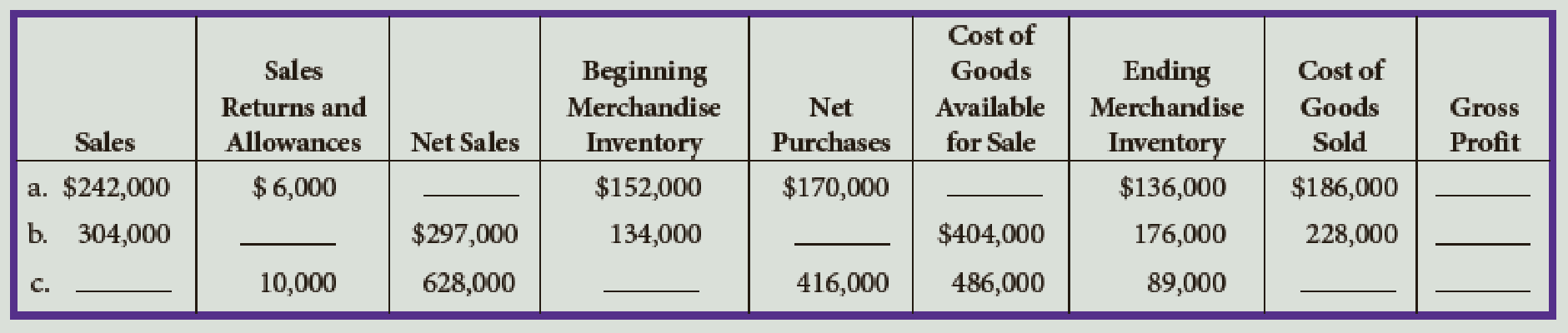Chapter 12, Problem 1E

Chapter
Section
Textbook Problem

Calculate the missing items in the following:To determine

Compute the missing items for (a), (b), and (c).

Explanation

Gross profit: The excess of price of merchandise sold over the cost of merchandise sold is referred to as gross profit.

Formula to compute gross profit:

Gross profit = Net sales – Cost of goods sold

 Details Amount ($) Amount ($) Amount ($) Amount ($) Sales $XXX Less: Sales returns and allowances$XXX Less: Sales discounts XXX XXX Net sales $XXX Cost of goods sold: Beginning merchandise inventory$XXX Purchases $XXX Less: Purchase returns and allowances$XXX Less: Purchases discounts XXX XXX Net purchases XXX Add: Freight-in XXX Cost of goods purchased XXX Cost of goods available for sale XXX Less: Ending merchandise inventory XXX Cost of goods sold XXX Gross profit \$XXX

Table (1)

Compute the missing items for (a)

Still sussing out bartleby?

Check out a sample textbook solution.

See a sample solution

The Solution to Your Study Problems

Bartleby provides explanations to thousands of textbook problems written by our experts, many with advanced degrees!

Get Started